# Analytical chemistry_Instrumentation_Introduction

22 de Aug de 2016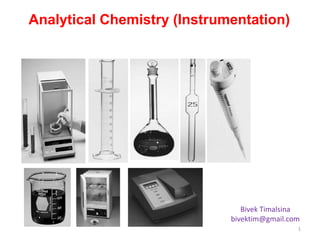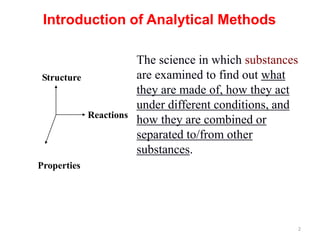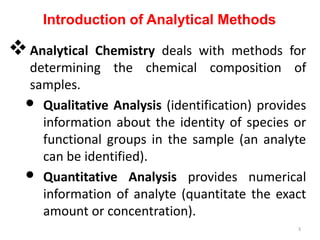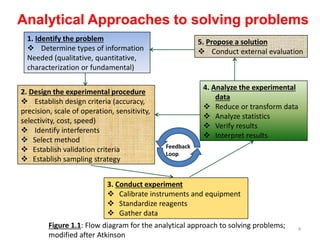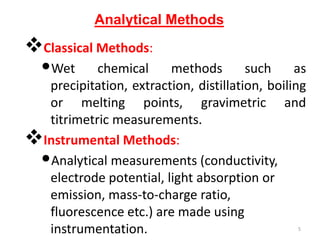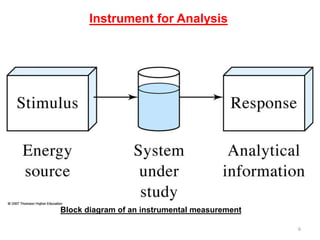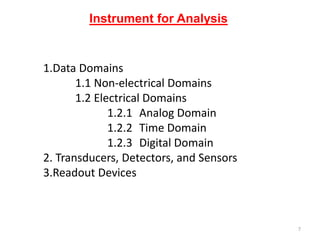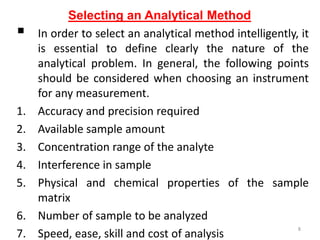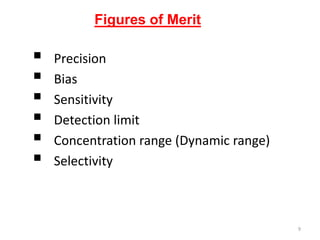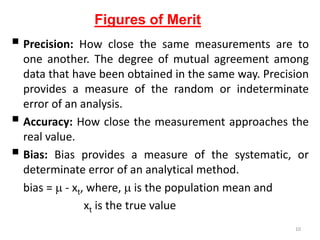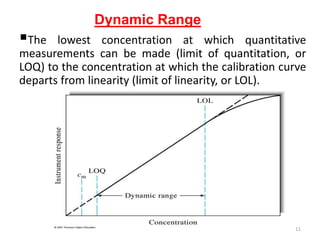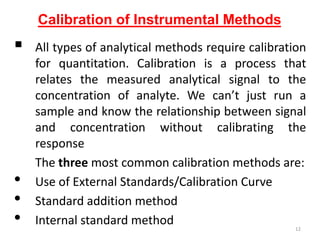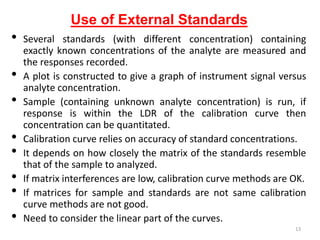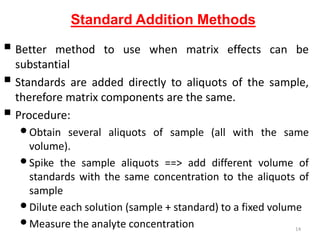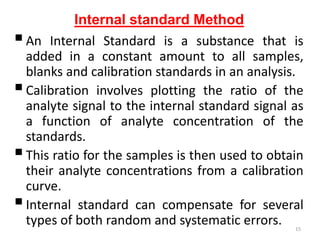1 de 15

### Analytical chemistry_Instrumentation_Introduction

1. Analytical Chemistry (Instrumentation) Bivek Timalsina bivektim@gmail.com 1
2. Introduction of Analytical Methods Structure Reactions Properties The science in which substances are examined to find out what they are made of, how they act under different conditions, and how they are combined or separated to/from other substances. 2
3. Analytical Chemistry deals with methods for determining the chemical composition of samples. • Qualitative Analysis (identification) provides information about the identity of species or functional groups in the sample (an analyte can be identified). • Quantitative Analysis provides numerical information of analyte (quantitate the exact amount or concentration). Introduction of Analytical Methods 3
4. Analytical Approaches to solving problems 1. Identify the problem  Determine types of information Needed (qualitative, quantitative, characterization or fundamental) 5. Propose a solution  Conduct external evaluation 2. Design the experimental procedure  Establish design criteria (accuracy, precision, scale of operation, sensitivity, selectivity, cost, speed)  Identify interferents  Select method  Establish validation criteria  Establish sampling strategy 4. Analyze the experimental data  Reduce or transform data  Analyze statistics  Verify results  Interpret results 3. Conduct experiment  Calibrate instruments and equipment  Standardize reagents  Gather data Feedback Loop Figure 1.1: Flow diagram for the analytical approach to solving problems; modified after Atkinson 4
5. Analytical Methods Classical Methods: •Wet chemical methods such as precipitation, extraction, distillation, boiling or melting points, gravimetric and titrimetric measurements. Instrumental Methods: •Analytical measurements (conductivity, electrode potential, light absorption or emission, mass-to-charge ratio, fluorescence etc.) are made using instrumentation. 5
6. Instrument for Analysis Block diagram of an instrumental measurement 6
7. Instrument for Analysis 1.Data Domains 1.1 Non-electrical Domains 1.2 Electrical Domains 1.2.1 Analog Domain 1.2.2 Time Domain 1.2.3 Digital Domain 2. Transducers, Detectors, and Sensors 3.Readout Devices 7
8.  In order to select an analytical method intelligently, it is essential to define clearly the nature of the analytical problem. In general, the following points should be considered when choosing an instrument for any measurement. 1. Accuracy and precision required 2. Available sample amount 3. Concentration range of the analyte 4. Interference in sample 5. Physical and chemical properties of the sample matrix 6. Number of sample to be analyzed 7. Speed, ease, skill and cost of analysis Selecting an Analytical Method 8
9.  Precision  Bias  Sensitivity  Detection limit  Concentration range (Dynamic range)  Selectivity Figures of Merit 9
10.  Precision: How close the same measurements are to one another. The degree of mutual agreement among data that have been obtained in the same way. Precision provides a measure of the random or indeterminate error of an analysis.  Accuracy: How close the measurement approaches the real value.  Bias: Bias provides a measure of the systematic, or determinate error of an analytical method. bias =  - xt, where,  is the population mean and xt is the true value Figures of Merit 10
11. The lowest concentration at which quantitative measurements can be made (limit of quantitation, or LOQ) to the concentration at which the calibration curve departs from linearity (limit of linearity, or LOL). Dynamic Range 11
12.  All types of analytical methods require calibration for quantitation. Calibration is a process that relates the measured analytical signal to the concentration of analyte. We can’t just run a sample and know the relationship between signal and concentration without calibrating the response The three most common calibration methods are: • Use of External Standards/Calibration Curve • Standard addition method • Internal standard method Calibration of Instrumental Methods 12
13. • Several standards (with different concentration) containing exactly known concentrations of the analyte are measured and the responses recorded. • A plot is constructed to give a graph of instrument signal versus analyte concentration. • Sample (containing unknown analyte concentration) is run, if response is within the LDR of the calibration curve then concentration can be quantitated. • Calibration curve relies on accuracy of standard concentrations. • It depends on how closely the matrix of the standards resemble that of the sample to analyzed. • If matrix interferences are low, calibration curve methods are OK. • If matrices for sample and standards are not same calibration curve methods are not good. • Need to consider the linear part of the curves. Use of External Standards 13
14.  Better method to use when matrix effects can be substantial  Standards are added directly to aliquots of the sample, therefore matrix components are the same.  Procedure: •Obtain several aliquots of sample (all with the same volume). •Spike the sample aliquots ==> add different volume of standards with the same concentration to the aliquots of sample •Dilute each solution (sample + standard) to a fixed volume •Measure the analyte concentration Standard Addition Methods 14
15. An Internal Standard is a substance that is added in a constant amount to all samples, blanks and calibration standards in an analysis. Calibration involves plotting the ratio of the analyte signal to the internal standard signal as a function of analyte concentration of the standards. This ratio for the samples is then used to obtain their analyte concentrations from a calibration curve. Internal standard can compensate for several types of both random and systematic errors. Internal standard Method 15